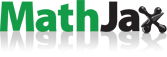# Adding MathJAX with LaTeX and MathML support to Jekyll

Pages, Numbers, and Keynote gots updates today with LaTeX and MathML support, and it reminded me that I wanted to add to my blog here. I always wanted to do this ever since I saw what was I think Dr. Drang’s MathJax post. Enter MathJax!## Configuring jekyll

Heavily borrowing from Adding MathJax to a GitHub Pages Jekyll Blog, it was a quick task to add. The main changes to repo:

_includes/mathjax.html
assets/js/MathJaxLocal.js
_layouts/posts.html


The first two are new files12 including the necessary javascript code and customization for MathJax. And the _layouts/posts.html edit is to embed the javascript into the jekyll-rendered post page.

modified   _layouts/post.html
@@ -2,6 +2,8 @@
layout: default
---

+{% include mathjax.html %}
+

<article class="post">
<h1>Adding MathJAX with LaTeX and MathML support to Jekyll</h1>


And in the Front Matter of post markdowns, on posts where mathjax is to be enabled:

[...]
mathjax: true
[...]
---


## Examples

These are my favorite part.

#### Newton’s law of universal gravitation

$F = G \frac{m_1 m_2} {r^2}$
$$F = G \frac{m_1 m_2} {r^2}$$


#### Einstein field equations

$R_{\mu \nu} - \frac{1} {2}Rg_{\mu \nu} + \Lambda g_{\mu \nu} = \frac{8\pi G} {c^4}T_{\mu \nu}$
$$R_{\mu \nu} - \frac{1} {2}Rg_{\mu \nu} + \Lambda g_{\mu \nu} = \frac{8\pi G} {c^4}T_{\mu \nu}$$

Published on June 15, 2018